Matrix elements between nonorthogonal Slater determinants represent an essential component of many emerging electronic structure methods. However, evaluating nonorthogonal matrix elements is conceptually and computationally harder than their orthogonal counterparts. While several different approaches have been developed, these are predominantly derived from the first-quantized generalized Slater–Condon rules and usually require biorthogonal occupied orbitals to be computed for each matrix element. For coupling terms between nonorthogonal excited configurations, a second-quantized approach such as the nonorthogonal Wick’s theorem is more desirable, but this fails when the two reference determinants have a zero many-body overlap. In this contribution, we derive an entirely generalized extension to the nonorthogonal Wick’s theorem that is applicable to all pairs of determinants with nonorthogonal orbitals. Our approach creates a universal methodology for evaluating any nonorthogonal matrix element and allows Wick’s theorem and the generalized Slater–Condon rules to be unified for the first time. Furthermore, we present a simple well-defined protocol for deriving arbitrary coupling terms between nonorthogonal excited configurations. In the case of overlap and one-body operators, this protocol recovers efficient formulas with reduced scaling, promising significant computational acceleration for methods that rely on such terms.

Matrix elements between nonorthogonal Slater determinants are increasingly common in emerging electronic structure methods. For example, capturing strong correlation using a linear combination of nonorthogonal Slater determinants is a relatively old idea1–4 that has seen a renaissance in the past decade.5–14 Similarly, nonorthogonal matrix elements arise in projected Hartree–Fock methods,15,16 while the combination of geminal-based nonorthogonal functions is an area of ongoing research.17 In each method, the nonorthogonality of different determinants can capture strong static correlation effects by breaking and restoring symmetries of the Hamiltonian,15 or it can provide a quasi-diabatic representation of dominant electronic configurations.5,9 Alternatively, multiple wave functions built from different orbitals arise in orbital-optimized excited states identified through methods such as ΔSCF,18–21 excited-state mean-field theory,22,23 or the complete active space self-consistent field (SCF).24,25 In these cases, orbital optimization can significantly improve predictions of charge transfer excitations, but nonorthogonal matrix elements are required for inter-state coupling terms such as oscillator strengths.

A variety of different approaches have been developed for the efficient evaluation of nonorthogonal matrix elements,26–30 which are predominantly derived from Löwdin’s general formula.31 The most popular framework in quantum chemistry is the generalized Slater–Condon rules,27,32 where biorthogonal occupied orbitals are constructed33,34 and a modified form of the Slater–Condon rules35 is applied depending on the number of zero-overlap orbital pairs in the biorthogonal basis. This approach is applicable to any pair of determinants but requires the diagonalization of the occupied orbital overlap matrix each time. In contrast, the development of many-body correlation methods using orthogonal determinants has greatly benefited from the second-quantized Wick’s theorem.36 While a nonorthogonal variant of Wick’s theorem exists, it is limited to determinants that have a non-zero many-body overlap and is not applicable if there are zero-overlap orbital pairs in the biorthogonal basis.37,38 This limitation arises because the Thouless theorem,39 used to relate two nonorthogonal determinants via an exponential transformation, breaks down when the two determinants have a zero many-body overlap. As a result, the nonorthogonal Wick’s theorem has seen only limited use in quantum chemistry.16,40,41

Computationally efficient nonorthogonal matrix elements become increasingly important in methods that use orthogonally excited configurations from nonorthogonal reference determinants. For example, including post-NOCI dynamic correlation in methods such as perturbative NOCI-MP242–44 and NOCI-PT2,14 or nonorthogonal multireference CI,3,16,41,45 requires overlap, one-body, or two-body coupling terms between excitations from nonorthogonal determinants. The number of nonorthogonal matrix elements therefore grows rapidly, and repeated biorthogonalization of the occupied orbitals becomes prohibitively expensive. In principle, the nonorthogonal Wick’s theorem could allow these matrix elements to be evaluated using only biorthogonal reference orbitals, but until now, this requires the reference determinants to have a strictly non-zero overlap.

In this contribution, we derive an entirely generalized nonorthogonal form of Wick’s theorem that applies to any pair of determinants with nonorthogonal orbitals, even if the overall determinants have a zero overlap. This new framework, which we call the “extended nonorthogonal Wick’s theorem,” provides the most general approach for deriving matrix elements using second-quantization, allowing Wick’s theorem and the generalized Slater–Condon rules to be unified for the first time.

One particular advantage of our approach is that it allows all matrix elements between excited configurations from a pair of nonorthogonal determinants to be derived using a single well-defined protocol. While some of the resulting expressions have previously been derived for various bespoke applications (see, e.g., Refs. 6, 42, and 45), these have often relied on the properties of matrix determinants to account for orbital excitations. Instead, we present a unifying theory that can recover all of these results and is automatically applicable in cases where the reference determinants have a zero overlap. Furthermore, we show how evaluating intermediates for a given pair of determinants can reduce the scaling of overlap and one-body coupling terms between excited configurations to $O1$. These particular non-orthogonal matrix elements then become almost as straightforward as the orthogonal Slater–Condon rules or Wick’s theorem, promising considerable acceleration for methods that rely on such terms.

To derive our generalized nonorthogonal matrix elements, we first define our notation in Sec. II. In Sec. III, we extend Thouless theorem39 to the case where the two determinants have nonorthogonal orbitals and a zero many-body overlap. Section IV combines this extended Thouless transformation with Wick’s theorem to create a generalized protocol for evaluating nonorthogonal matrix elements using second-quantization. We then illustrate the application of this approach by re-deriving the generalized Slater–Condon rules for one- and two-body operators in Sec. V. Finally, in Sec. VI, we extend our framework to the matrix elements between excited configurations and show how $O1$ scaling can be achieved for overlap and one-body operators.

We will consider matrix elements between the two determinants $Φw$ and $Φx$. Each determinant is constructed from a bespoke set of molecular orbitals (MOs), represented in terms of the atomic spin-orbital basis functions $χμ$ as

$Φpw=∑μχμ C⋅pμ⋅w.$
(1)

Here, we employ the nonorthogonal tensor notation of Head-Gordon et al.46 to explicitly keep track of any required overlap matrices. Occupied MOs are indexed as i, j, k, etc., virtual MOs are indexed as a, b, c, etc., and any general MO is indexed as p, q, r, etc. We emphasize that the MOs are orthogonal within each Slater determinant but are nonorthogonal between the different determinants.

In second-quantization, the N-electron determinant $Φw$ is defined as

$Φw=∏i=1N bi†x−,$
(2)

where $−$ is the physical vacuum and the molecular orbital creation operators $bi†x$ satisfy the standard fermionic anticommutation rules.47 Using the expansion [Eq. (1)], the MO creation and annihilation operators can be represented in terms of the covariant atomic spin-orbital creation $aμ†$ and annihilation aμ operators as

$bp†x=∑μaμ† C⋅pμ⋅w and bpw=∑μ(C*w)p⋅⋅μaμ.$
(3)

The covariant atomic spin-orbital operators have only one non-zero anticommutator,47

$[aν†,aμ]+=gμν,$
(4)

where gμν = ⟨χμ|χν⟩ defines the corresponding covariant metric tensor (overlap matrix).46

Throughout this paper, we will need to express the atomic spin-orbital creation and annihilation operators in terms of the MO creation and annihilation operators using the inverse of Eq. (3),

$aμ†=∑pσbp†x (C*w)p⋅⋅σgσμ,$
(5a)
$aμ=∑pσgμσ(Cw)⋅pσ⋅ bpw.$
(5b)

To avoid the introduction of overlap matrices throughout our expressions, we will often use the contravariant atomic spin-orbital operators $(aμ)†$ and aμ defined as

$(aν)†=∑μaμ† gμν and aν=∑μgνμaμ,$
(6)

where gμν is the contravariant metric tensor corresponding to the inverse covariant overlap matrix,46 i.e.,

$gμν=(g−1)μν.$
(7)

If the AO basis is overcomplete, this contravariant metric tensor becomes the pseudo-inverse of the covariant overlap matrix. Note that the anticommutator of these contravariant atomic spin-orbital operators is

$[(aν)†,aμ]+=gμν.$
(8)

The conventional form of Thouless theorem allows two nonorthogonal determinants to be related by an exponential operator of single excitations as39

$Φw=exp(Z)Φx⟨Φx|Φw⟩.$
(9)

To derive the single excitation operator $Z$, the occupied orbitals can be transformed to a biorthogonal basis using Löwdin pairing33,34 such that

$∑μν(C̃*x)i⋅⋅μ gμνC̃⋅jν⋅w=S̃ixwδij.$
(10)

The virtual–occupied and virtual–virtual blocks of the biorthogonalized overlap matrix become

$∑μν(C̃*x)a⋅⋅μ gμνC̃⋅pν⋅w=S̃apxw,$
(11)

and the transformed molecular orbital creation and annihilation operators are given as

$b̃p†w=∑μaμ† C̃⋅pμ⋅w and b̃pw=∑μ(C̃*w)p⋅⋅μ aμ.$
(12)

The single excitation operator in Eq. (9) is then defined as

$Z=∑iaZaixw b̃a†x b̃ix,$
(13)

with the xwZai matrix elements given by

$Zaixw=∑μν(C̃*x)a⋅⋅μ gμν (C̃w)⋅iν⋅1S̃ixw.$
(14)

A brief derivation of this result can be found in  Appendix A.

Unfortunately, this exponential representation relies on the strict nonorthogonality of the two determinants ⟨xΦ$|w$Φ⟩ ≠ 0; in other words, it is not applicable to a pair of determinants that are orthogonal but contain mutually nonorthogonal orbitals. Our first step is therefore a generalization of the Thouless transformation to the case where ⟨xΦ$|w$Φ⟩ = 0.

We begin in the biorthogonal basis identified through Löwdin pairing, with orbital coefficients satisfying Eq. (10). For a general pair of nonorthogonal orbitals, it is possible for orbital pairs to have a zero-overlap in the biorthogonal basis, where $S̃ixw=0$. Taking the case with m zero overlaps between orbitals k1, …, km, we construct “reduced” determinants by removing the electrons in these zero-overlap orbitals to give

$Φk1⋯kmx=b̃kmx⋯b̃k1xΦx,$
(15a)
$Φk1⋯kmw=b̃kmw⋯b̃k1wΦw.$
(15b)

These reduced determinants are strictly nonorthogonal with the non-zero reduced overlap defined as

$S̃xw=⟨Φk1⋯kmx|Φk1⋯kmw⟩=∏{i|xwS̃i≠0}S̃ixw.$
(16)

Therefore, the Thouless transformation can now be applied to these reduced determinants to give

$Φk1⋯kmw=exp(Z̃)Φk1⋯kmxS̃xw.$
(17)

Here, we have introduced the reduced single excitation operator $Z̃$ that only contains excitations from occupied orbitals with a non-zero overlap as

$Z̃=∑{i|xwS̃i≠0}∑aZaixw b̃a†x b̃ix.$
(18)

The full N-electron determinants are then related through second-quantization as

$Φw=b̃k1†w⋯b̃km†w⁡exp(Z̃)b̃kmx⋯b̃k1xΦxS̃xw.$
(19)

Equation (19) can be further simplified by exploiting the commutativity relation $[Z̃,b̃kx]=0$ to shift the $exp(Z̃)$ operator to the far right-hand side, giving

$Φw=b̃k1†w⋯b̃km†wb̃kmx⋯b̃k1x⁡exp(Z̃)ΦxS̃xw.$
(20)

We can then introduce single-electron excitation operators $z^k$ for the zero-overlap orbitals as

$z^k=b̃k†wb̃kx=∑p∑μν(C̃*x)p⋅⋅μgμν(C̃w)⋅kν⋅b̃p†xb̃kx=∑aS̃akxw b̃a†xb̃kx,$
(21)

where we have exploited the biorthogonality and zero-overlap of the occupied orbitals such that $S̃ikxw=0$ for all i. The commutativity of these single excitation operators with the $b̃kx$ annihilation operators leads to the simplified relationship

$Φw=∏{k|xwS̃k=0}z^k⁡exp(Z̃)ΦxS̃xw.$
(22)

Introducing the relationship $z^k=exp(z^k)−1$ allows Eq. (22) to be expanded as

$Φw=∏{k|xwS̃k=0}exp(z^k)−1expZ̃ΦxS̃xw.$
(23)

Expanding the product of $(exp(z^k)−1)$ terms then leads to a sum of exponential transformations where every combination of the zero-overlap single excitation operators $z^k$ is either included or excluded with an appropriate phase factor, giving

$Φw=∑n=0m(−1)(m−n)∑CnexpZ̃CnΦxS̃xw.$
(24)

Here, we have introduced the compound index $Cn$ to denote a particular combination of n out of m zero-overlap orbitals, while the superscript notation $Z̃Cn$ indicates which particular zero-overlap excitations are included in the corresponding operator, i.e.,

$Z̃Cn=Z̃+∑k∈Cnz^k.$
(25)

We refer to this transformation in Eq. (24) as the “extended Thouless transformation.” To explicitly illustrate its application, the case of two zero-overlaps in orbital pairs k1 and k2 leads to

$Φw=S̃xwexpZ̃(k1,k2)−expZ̃(k1)−expZ̃(k2)+expZ̃Φx,$
(26)

where $Z̃(k1,k2)=Z̃+z^k1+z^k2$ and $Z̃(k1)=Z̃+z^k1$.

Efficiently deriving matrix elements using the conventional Wick’s theorem requires the introduction of contractions, defined for two creation or annihilation operators xbp and xbq as

where {xbpxbq} represents a normal-ordered operator string with respect to the reference Fermi vacuum ⟨xΦ|⋯|xΦ⟩.36 The only non-zero contractions between creation and annihilation operators with respect to this symmetric Fermi vacuum are

Through Wick’s theorem, the Fermi vacuum expectation of an operator product is given by the sum over all fully contracted products of operators, e.g.,

Using the extended Thouless transformation, we can now extend the nonorthogonal Wick’s theorem37,38,48 to derive matrix elements between any pair of determinants with mutually nonorthogonal orbitals. In what follows, we will consider the contravariant atomic spin-orbital operators (see Sec. II) to avoid large numbers of overlap matrices in our expressions. The matrix elements for general operators expressed in the atomic spin-orbital basis requires the evaluation of terms containing a string of creation and annihilation operators, such as $⟨Φx|(aμ)†(aν)†⋯aσaτ|Φw⟩$. Applying the extended Thouless transformation leads to the linear combination

$⟨Φx|(aμ)†(aν)†⋯aσaτ|Φw⟩ =S̃xw∑n=0m(−1)(m−n)∑Cn⟨Φx|(aμ)†(aν)†⋯aσaτ⁡expZ̃Cn|Φx⟩.$
(30)

To evaluate each constituent matrix element for the combinations $Cn$, we follow the approach described in Refs. 38 and 40 and introduce a similarity-transformed set of spin-orbital creation and annihilation operators as

$(d[Cn]μ)†=exp−Z̃Cn(aμ)†⁡expZ̃Cn,$
(31a)
$d[Cn]μ=exp−Z̃Cnaμ⁡expZ̃Cn.$
(31b)

These operators clearly depend on the particular combination $Cn$ of included zero-overlap single excitation operators. Expanding the similarity transformation as

$exp−Z̃Cnaμ⁡expZ̃Cn=aμ−[Z̃Cn,aμ],$
(32)

and similarly for $(aμ)†$, leads to the explicit forms

$(d[Cn]μ)†=∑ib̃i†x(C̃*x)i⋅⋅μ+∑ab̃a†x(C̃*x)a⋅⋅μ −∑{i|xwS̃i≠0}Zaixw(C̃*x)i⋅⋅μ−∑k∈CnS̃akxw(C̃*x)k⋅⋅μ,$
(33a)
$d[Cn]μ=∑i(C̃x)⋅iμ⋅b̃ix+∑a∑{i|xwS̃i≠0}(C̃x)⋅aμ⋅ Zaixwb̃ix +∑k∈Cn(C̃x)⋅aμ⋅ S̃akxw b̃kx+(C̃x)⋅aμ⋅ b̃ax.$
(33b)

An explicit derivation of these relationships can be found in  Appendix B. Exploiting the relationship

$Φxexp−Z̃Cn=Φx$
(34)

and the resolution of the identity

$expZ̃Cnexp−Z̃Cn=I$
(35)

then allows the constituent matrix elements within Eq. (30) to be expressed as

$⟨Φx|(aμ)†(aν)†⋯aσaτ⁡expZ̃Cn|Φx⟩ =⟨Φx|(d[Cn]μ)†(d[Cn]ν)†⋯d[Cn]σd[Cn]τ|Φx⟩.$
(36)

The extended Thouless transformation essentially converts the nonorthogonal matrix element with an asymmetric Fermi vacuum ⟨xΦ|⋯$|w$Φ⟩ to a transformed matrix element with respect to symmetric Fermi vacuum ⟨xΦ|⋯|xΦ⟩. Since the transformed operators $d[Cn]μ$ and $(d[Cn]μ)†$ are expressed purely in terms of the $b̃px$ creation and $b̃p†x$ annihilation operators, with respect to the ⟨xΦ|⋯|xΦ⟩ vacuum, their non-zero contractions with respect to ⟨xΦ|⋯|xΦ⟩ can be derived by combining Eqs. (28) and (33) to give

where we have introduced the general notation

$Pkνμxw=(C̃w)⋅kν⋅(C̃*x)k⋅⋅μ,$
(38a)
$Pνμxw=∑{k|xwS̃k=0}Pkνμxw,$
(38b)
$Wνμxw=∑{i|xwS̃i≠0}(C̃w)⋅iν⋅1S̃ixw(C̃*x)i⋅⋅μ,$
(38c)
$Mνμxw=Pνμxx+Pνμxw+Wνμxw.$
(38d)

These expressions closely resemble the co-density matrices in Ref. 5, and their derivation can be found in  Appendix C.

The overall matrix element $⟨Φx|(aμ)†(aν)†⋯aσaτ|Φw⟩$ requires the derivation of contractions between the aμ and $(aμ)†$ atomic spin-orbital operators rather than the transformed $d[Cn]μ$ and $(d[Cn]μ)†$ operators. To show how a general string of operators can be evaluated using the contractions defined in Eqs. (37a) and (37b), we first demonstrate the derivation of the one-body co-density matrix element

$Γ1νμxw=⟨Φx|(aμ)†aν|Φw⟩.$
(39)

Assuming that some form of Wick’s theorem can be derived, we expect this matrix element to be represented by the single contraction

Taking the most general case with m zero-overlap orbitals, the extended Thouless transformation leads to

Reversing the order of summation over k and $Cn$ yields

The first term in square brackets $∑Cn1$ can be recognized as the total number of ways to pick n orbitals from the m zero-overlap orbitals, given by $mn$. Similarly, the second term in square brackets $∑Cn̸∋ k1$ is the total number of ways to pick n orbitals from the m − 1 zero-overlap orbitals that remain when orbital k is excluded, given by $m−1n$. These combinatorial expansions allow Eq. (42) to be expressed as

Here, we note that there are no ways to exclude an orbital k when all zero-orbital overlaps are included in the complete combination $Cm$. Exploiting the binomial expansion

$∑n=0y(−1)(y−n)yn=δ0,y$
(44)

then leads to the closed-form expression

The reduced overlap $S̃xw$ will be a prefactor for every matrix element between these nonorthogonal determinants. The remaining terms can then be used to define “fundamental contractions” for second-quantization operators with respect to the asymmetric Fermi vacuum ⟨xΦ|⋯$|w$Φ⟩. The form of these contractions depends on the number of zero-overlap orbitals m, and we define the first fundamental contraction as

Similarly, the second fundamental contraction can be identified as

Crucially, we emphasize that these fundamental contractions are defined with respect to the asymmetric Fermi vacuum ⟨xΦ|⋯$|w$Φ⟩.

Next, we show how the fundamental contractions can be combined to derive matrix elements for longer products of creation and annihilation operators. As an example, consider the two-body reduced co-density matrix element, defined as

$Γ2τμσνxw=⟨Φx|(aμ)†(aν)†aσaτ|Φw⟩.$
(48)

Applying Wick’s theorem, this matrix element should be given by the sum of the two contractions,

Note that the second term in this expression carries a phase of −1 from the intersection of the contraction lines, representing the fundamental parity of fermionic operators.36 Taking the first contraction as an example, we apply the extended Thouless transformation and the transformed contractions defined in Eqs. (37a) and (37b) to give

Once again, the reduced overlap $S̃xw$ appears as an overall prefactor. The order of summation over the k1, k2 indices and $Cn$ can then be swapped to give

The third term in square brackets $∑Cn̸∋k1,k21$ is simply the number of ways to pick n orbitals from the m − 2 zero-overlap orbitals that remain when orbitals k1 and k2 are removed, given by $m−2n$. Applying the binomial expansion Eq. (44) in an analogous way to the single contraction leads to the closed form

A similar expression can be derived for the second contraction as

which, analogously with the orthogonal case, will carry a −1 phase factor from the intersection of the contraction lines. Combining Eqs. (52) and (53), with their associated phase factors, yields the full expression for the two-body reduced co-density matrix elements with m zero-overlap orbitals as

$⟨Φx|(aμ)†(aν)†aσaτ|Φw⟩=S̃xwMτμxw Mσνxw− Mσμxw MτνwxMτνwxMτνwx, m=0S̃xwPτμxw Mσνxw+ Mτμxw Pσνxw−Pσμxw Mτνxw−Mσμxw Pτνxw, m=1S̃xwPτμxw Pσνxw−Pσμxw Pτνxw, m=20, m≥3.$
(54)

To simplify the derivation of even longer operator strings, we note that the two-body matrix elements in Eq. (52) can be factorized into the product of two fundamental contractions with individual m1 and m2 values under the constraint m1 + m2 = m, giving

This factorization can be extended for a general product of contractions with each mi restricted to the values 0 or 1 for the overall product to be non-zero. We can therefore define an intuitive approach for extending Wick’s theorem to generalized nonorthogonal matrix elements as follows:

1. Construct all fully contracted combinations of the operator product with the associated phase factors.

2. For each term, sum every possible way to distribute m zeros among the contractions such that ∑imi = m.

3. For every set of {mi} in each term, construct the relevant contribution as a product of fundamental contractions depending on whether each contraction has mi = 0 or 1.

4. Multiply the final combined expression with the reduced overlap $S̃xw$.

These rules and contractions therefore allow any matrix element to be evaluated with respect to the asymmetric Fermi vacuum ⟨xΦ|⋯$|w$Φ⟩ for nonorthogonal orbitals, regardless of whether the many-body determinants are orthogonal or not. As a result, our formulation is the most flexible form of Wick’s theorem and reduces to the previous nonorthogonal37,38,48 or orthogonal36 variants under suitable restrictions on the MO coefficients. We refer to this approach as the “extended nonorthogonal Wick’s theorem.” In Secs. V and VI, we will show how these steps can be applied to recover the generalized Slater–Condon rules32 and to derive matrix elements between excited configurations with respect to the nonorthogonal reference determinants.

The generalized Slater–Condon rules provide a first-quantized approach for evaluating matrix elements between nonorthogonal determinants. A detailed description of these rules, their derivation, and their application can be found in Ref. 32. However, to the best of our knowledge, the generalized Slater–Condon rules have never previously been derived though a fully second-quantized framework. In this section, we will show how these rules can be recovered using the extended nonorthogonal Wick’s theorem.

Consider first the one-body operator

$f^=∑μνfμν(aμ)†aν,$
(56)

with the corresponding matrix element

$⟨Φx|f^|Φw⟩=∑μνfμν⟨Φx|(aμ)†aν|Φw⟩.$
(57)

Applying the extended nonorthogonal Wick’s theorem yields only one non-zero contraction to give

Substituting the fundamental contraction [Eq. (46)] and considering the possible values of m immediately yield the one-body generalized Slater–Condon rules32 in the form presented in Ref. 5 as

$⟨Φx|f^|Φw⟩=S̃xw∑μνfμν(Wxw)νμ no zerosS̃xw∑μνfμν(Pkxw)νμ one zero(k)0 otherwise.$
(59)

Here, we note that xwMνμ = xwWνμ when there are no zero-overlap orbitals, while $Pνμxw=Pkνμxw$ for one-zero overlap in orbital k. The matrices xwM and xwPk correspond, respectively, to the weighted and unweighted co-density matrices discussed in Ref. 5.

Next, consider a general two-body operator $v^$ defined in second-quantization as

$v^=∑μνστvμντσ(aμ)†(aν)†aσaτ,$
(60)

with the two-electron integrals in the spin-orbital basis defined as

$vμντσ=⟨χμχν|v^|χτχσ⟩.$
(61)

The corresponding matrix element is given by

$⟨Φx|v^|Φw⟩=∑μνστvμντσ⟨Φx|(aμ)†(aν)†aσaτ|Φw⟩.$
(62)

Recognizing the $⟨Φx|(aμ)†(aν)†aτaσ|Φw⟩$ term as the two-body reduced co-density matrix derived in Eq. (54), we immediately recover

$⟨Φx|v^|Φw⟩=S̃xw ∑μνστvμντσMτμxw Mσνxw− Mσμxw Mτνxw, m=0S̃xw ∑μνστvμντσPτμxw Mσνxw+ Mτμxw Pσνxw−Pσμxw Mτνxw−Mσμxw Pτνxw, m=1S̃xw ∑μνστvμντσPτμxw Pσνxw−Pσμxw Pτνxw, m=20, m≥3.$
(63)

Here, note that the m = 1 terms each contain two terms with the single zero-overlap in either the first or second contraction. Exploiting the symmetry $v$μντσ = $v$νμστ and the identity

$Pτμxw Pσνxw−Pσμxw Pτνxw=∑k1≠k2Pk1τμxw Pk2σνxw−Pk1σμxw Pk2τνxw$
(64)

then allows the two-body generalized Slater–Condon rules32 to be recovered as

$⟨Φx|v^|Φw⟩=S̃xw ∑μνστvμντσWτμxw Wσνxw−Wσμxw Wτνxw, no zeros2 S̃xw ∑μνστvμντσPkτμxw Wσνxw−Pkσμxw Wτνxw, one zero(k)2 S̃xw ∑μνστvμντσPk1τμxw Pk2σνxw−Pk1σμxw Pk2τνxw, two zeros(k1,k2)0, otherwise.$
(65)

Note that for the m = 1 case with one zero-overlap in orbital k, we have exploited the identities $Pkτμxw Pkσνxx=Pkσμxw Pkτνxx$ and $Pkτμxw Pkσνxw=Pkσμxw Pkτνxw$ to introduce the simplification xwMxwW.

While re-deriving the generalized Slater–Condon rules provides an important verification of the extended nonorthogonal Wick’s theorem, it does not provide any computational advantage over the original framework. On the contrary, the primary focus of our new framework involves deriving matrix elements between excited configurations from a pair of nonorthogonal determinants, e.g., $⟨Φij…ab…x|⋯|Φkl…cd…w⟩$. Terms of this form arise in perturbative corrections to NOCI,14,42–44 the NOCI–CIS approach for core excitations,8,49 and the evaluation of ⟨S2⟩ coupling terms in NOCI expansions.6 Furthermore, these nonorthogonal matrix elements will be required to evaluate inter-state coupling elements between orbital-optimized excited-state wave functions identified using excited-state mean-field theory22 or state-specific complete active space SCF.24

Until now, evaluating these matrix elements has required the direct application of the generalized Slater–Condon rules to each pair of excitations. This approach leads to significant computational costs associated with the biorthogonalization of the excited determinants, which scales as $ON3$ each time. In this section, we show how the extended nonorthogonal Wick’s theorem allows these matrix elements to be evaluated using only biorthogonalized reference determinants. When large numbers of coupling elements are required, avoiding the biorthogonalization of these excited configurations significantly reduces the overall computational cost. Furthermore, the cost of certain nonorthogonal matrix elements (including all one-body operators) becomes independent of the number of electrons or basis functions if additional intermediate matrix elements are computed for each pair of reference determinants.

In this section, we describe how the extended nonorthogonal Wick’s theorem can be applied to matrix elements between excited configurations. First, we discuss how the fundamental contractions described in Sec. IV C can be modified to an MO-based form that makes excited configurations easier to handle. We then illustrate the derivation of certain overlap, one-body, and two-body matrix elements between nonorthogonal excited configurations. The resulting expressions are entirely generalized for any pair of nonorthogonal reference determinants and can significantly reduce the computational scaling compared to a naïve application of the generalized Slater–Condon rules.

Evaluating matrix elements between two excited determinants will require the evaluation of the asymmetric contractions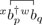with respect to the asymmetric Fermi vacuum ⟨xΦ|⋯|wΦ⟩. Expanding the molecular orbital creation and annihilation operators using Eq. (3) yields

Introducing the fundamental contractions for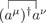and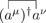defined in Eqs. (46) and (47), respectively, then reduces these expressions to different forms depending on the number of zero-overlaps mk associated with the contraction,

Here, we have defined the “screened” overlap terms

$Xqpwx=∑μνστ(C*w)q⋅⋅μ gμσ(Mx)στ gτν (Cx)⋅pν⋅,$
(68a)
$X¯qpwx=∑μνστ(C*w)q⋅⋅μ gμσ(Px)στ gτν (Cx)⋅pν⋅,$
(68b)

and

$Ypqxw=−Xpqxw−Spqxw,$
(69a)
$Ȳpqxw=−X¯pqxw,$
(69b)

with xwP and xwM defined in Eqs. (38b) and (38d), respectively, and the overlap element

$Spqwx=∑μν(C*w)p⋅⋅μ gμν (Cx)⋅qν⋅.$
(70)

Although the indexing notation used in Eq. (68a) may seem counterintuitive, we find that it helps to keep track of the bra and ket orbital coefficients in the screened overlap terms.

Crucially, the orbital coefficients used to evaluate these contractions do not need to be the same as those used to evaluate the xwM and xwP matrices. This feature is particularly advantageous as the excited configurations can be represented in terms of the original orbital basis while the xwM and xwP matrices are evaluated in the biorthogonal basis. As a result, only the reference determinants need to be biorthogonalized, and the remaining matrix elements are evaluated in terms of these screened overlap terms. Furthermore, the screened overlap elements are themselves one-body matrix elements that can be computed once for a given pair of determinants and stored, before being combined to evaluate more complicated matrix elements. With Nref determinants, the total cost of computing these intermediates therefore scales as $ONref2 n3$.

To take full advantage of these asymmetric contractions, the one- and two-body operators can also be represented in terms of one set of molecular orbitals as

$f^=∑pqfpqxbp†xbqx,$
(71a)
$v^=∑pqrsvpqrsxbp†xbq†xbsxbrx,$
(71b)

where we have defined the transformed matrix elements

$fpqx=∑μν(C*x)p⋅⋅μ fμν (Cx)⋅qν⋅$
(72)

and

$vpqrsx=∑μνστ(C*x)p⋅⋅μ(C*x)q⋅⋅ν vμνστ (Cx)⋅rσ⋅(Cx)⋅sτ⋅.$
(73)

Evaluating nonorthogonal matrix elements through the extended nonorthogonal Wick’s theorem then proceeds using similar steps outlined in Sec. IV E:

1. Assemble all fully contracted combinations of the asymmetric operator and excitation operator product and compute the corresponding phase factors.

2. For each term, sum every possible way to distribute m zeros among the contractions such that ∑imi = m.

3. For every set of {mi} in each term, construct the relevant contribution as a product of fundamental contractions defined in Eqs. (67a) and (67b) depending on whether each contraction has mi = 0 or 1.

4. Multiply the combined expression with the reduced overlap $S̃xw$ of the reference determinants.

Note that the number of zero-overlap orbitals in these expressions corresponds to the biorthogonalized reference determinants, not the excited configurations.

In Secs. IV B–IV E, we will illustrate this process through a series of typical nonorthogonal matrix elements. As we shall see below, evaluating the sum of every combination of zero-overlap indices assigned to each contraction quickly leads to complicated equations. However, we can derive the general structure of a matrix element for the case with no zero-overlap orbitals m = 0 and then recover the forms for different values of m by distributing the zero-overlap indices over each fundamental contraction. We will therefore focus on the parent equation with m = 0, which we refer to as the “canonical form” and denote using the notation ⟨⋯⟩0. Furthermore, any matrix element with more zero-overlap orbitals than the total number of contractions must be strictly zero.

First, we consider the overlap element between excited determinants. The simplest overlap matrix element involves only a single excitation $⟨Φx|Φiaw⟩$. For m zero-overlap orbitals in the reference determinants, this single excitation matrix element can be identified using one contraction as

Here, the canonical form is simply

$⟨Φx|Φiaw⟩0=S̃xw Xiaww,$
(75)

and there is only one way to assign one zero-overlap orbital to the single contraction. Note that the reduced overlap between the reference determinants remains a prefactor for the overall matrix elements.

Next, the overlap of two single excitations $⟨Φiax|Φjbw⟩$ can be evaluated as

The canonical form for this element is the m = 0 term

$⟨Φiax|Φjbw⟩0=S̃xwXjbwwXaixx+XjiwxYabxw.$
(77)

The m = 1 term is recovered by taking the sum of the two different ways to distribute one zero-overlap orbital to the two contractions, while there is only one way to distribute two zero-overlap orbitals for the m = 2 term.

As a third example, consider the double excitation overlap $⟨Φx|Φijabw⟩$, which can be evaluated as

In this case, the minus sign arises from the intrinsic −1 phase that results from the intersection of the contraction lines in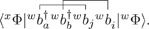The relevant canonical form of this matrix element is

$⟨Φx|Φijabw⟩0=S̃xwXiawwXjbww−XibwwXjaww,$
(79)

from which the terms for m = 1 and m = 2 can be derived. Notably, this form of $⟨Φx|Φijabw⟩$ has previously be derived for m = 0 in Refs. 42 and 45, but our derivation generalizes for any number of zero-overlap orbitals.

We now consider matrix elements for one-body operators of the form given in Eq. (71a). To further simplify the subsequent expressions, we can introduce intermediate matrices that account for partial contraction with the one-body operator. In particular, we introduce the partially contracted intermediate terms

$F0=∑pqfpqxXqpxx,$
(80a)
$[XFX]rsyw=∑pqXrpyxfpqxXqsxw,$
(80b)
$[XFY]rsyw=∑pqXrpyxfpqxYqsxw,$
(80c)
$[YFX]rsyw=∑pqYrpyxfpqxXqsxw,$
(80d)
$[YFY]rsyw=∑pqYrpyxfpqxYqsxw.$
(80e)

Note that the individual contraction in the F0 term, indicated by the X matrix, may correspond to a zero-overlap orbital pair, as indicated by the notation $F¯0$. The ye[XFX]rs (and similar) terms correspond to two contractions and can thus be assigned two zero overlap orbitals. The different possibilities of assigning these zero-overlap contractions is denoted as $[X¯FX]rsyw$ or $[XFX¯]rsyw$ and similarly for terms involving the Y contraction. Crucially, these intermediate values also correspond to orbital pairs and can be precomputed once for each pair of reference determinants, leading to a one-off computational cost that scales as $ONref2 n3$. As a result, the summation over the p, q indices is avoided for the subsequent evaluation of matrix elements between excited determinants, and the computational scaling of these one-body terms is the same as the overlap matrix elements.

To illustrate the application of this approach, we take the simplest one-body matrix element $⟨Φx|f^|Φiaw⟩$. For m zero-overlap orbitals in the reference determinants, this matrix element can be expanded as

Using the contractions defined in Eqs. (67a) and (67b), and the intermediate terms defined in Eq. (80), the canonical form for m = 0 is given as

$⟨Φx|f^|Φiaw⟩0=S̃xwF0Xiaww+[XFY]iaww.$
(82)

If F0, wwXia, and ww[XFY]ia are precomputed and stored once for the reference determinants, the subsequent cost of evaluating $⟨Φx|f^|Φiaw⟩$ is independent of the number of electrons or basis functions and scales as $O1$. From this canonical form, expressions for m = 1 and 2 (or higher) can be identified as

$⟨Φx|f^|Φiaw⟩=S̃xwF0Xiaww+[XFY]iaww, m=0S̃xwF¯0Xiaww+[X¯FY]iaww+F0X¯iaww+[XFȲ]iaww, m=1S̃xwF¯0X¯iaww+[X¯FȲ]iaww, m=20, m>2.$
(83)

Next, consider the coupling of two single excitations $⟨Φiax|f^|Φjbw⟩$, corresponding to the fully contracted terms

In this case, the canonical form is given by

$⟨Φiax|f^|Φjbw⟩0=S̃xwF0XaixxXjbww+F0XjiwxYabxw+[XFY]jbwwXaixx+[YFX]aixxXjbww+[YFY]abxwXjiwx−[XFX]jiwxYabxw.$
(85)

Again, the form for m zero-overlap orbitals can be recovered as the sum of every way to distribute the zero-overlap contractions over the X, Y, or F0 terms in each product. We omit the explicit form of these m > 0 expressions to maintain brevity.

Finally, consider the one-body coupling of a reference determinant and a double excitation

The corresponding canonical form for m = 0 is

$⟨Φx|f^|Φijabw⟩0=S̃xwF0XiawwXjbww−F0XibwwXjaww+[XFY]iawwXjbww+[XFY]jbwwXiaww−[XFY]jawwXibww−[XFY]ibwwXjaww,$
(87)

from which expressions for m > 0 can be obtained as described above.

Finally, we consider matrix elements for two-body operators, with the general form given in Eq. (71b). Again, we can define intermediate matrices in the orbital basis that can be pre-computed for a given pair of nonorthogonal determinants. First, we introduce analogs of the Coulomb and exchange matrices, respectively, defined as

$Jprx=∑qsvpqrsxXsqxx,Kpsx=∑qrvpqrsxXrqxx.$
(88)

Partially contracted intermediate matrices can then be defined as, e.g.,

$[X(J−K)X]rsyw=∑pqXrpyx(Jx−Kx)pqXqsxw,$
(89a)
$[Y(J−K)X]rsyw=∑pqYrpyx(Jx−Kx)pqXqsxw,$
(89b)
$[X(J−K)Y]rsyw=∑pqXrpyx(Jx−Kx)pqYqsxw,$
(89c)
$[Y(J−K)Y]rsyw=∑pqYrpyx(Jx−Kx)pqYqsxw,$
(89d)

with the constant value

$V0=∑pqrsvpqrsxXsqxxXrpxx−XrqxxXspxx.$
(90)

The one-off computational cost of evaluating each of these intermediate matrices is dominated by the cost of evaluating Eqs. (88) and (90) for each reference determinant, and so the overall scaling is $ONref n4$.

Like the one-body intermediate matrices, when the zero-overlap orbitals are distributed among the contractions, these zeros may be independently assigned to any of the X, Y, or (JK) terms, as each of these contain one contraction. For example, with m = 1, one must consider the sum of three terms given as, e.g.,

$[X¯(J−K)X]rsyw+[X(J¯−K¯)X]rsyw+[X(J−K)X¯]rsyw,$
(91)

where

$J¯prx=∑qsvpqrsxX¯sqxx$
(92a)
$K¯psx=∑qrvpqrsxX¯rqxx.$
(92b)

On the contrary, the V0 constant term corresponds to two contractions and can be assigned two zero-overlap orbitals. Noting the symmetry x$v$pqrs = x$v$qpsr, we denote these possibilities as

$V¯0=2∑pqrsvpqrsxX¯sqxxXrpxx−X¯rqxxXspxx,$
(93a)
$V¯¯0=∑pqrsvpqrsxX¯sqxxX¯rpxx−X¯rqxxX¯spxx.$
(93b)

Here, the factor of two in Eq. (93a) arises because the zero-overlap orbital can be assigned to either the first or second contraction to give the same result.

To illustrate the application of this approach for excited configurations, we first consider the two-body matrix element

Combining each contraction and exploiting the intermediates in Eqs. (89) and (90) yields the canonical form (m = 0) for this matrix element as

$⟨Φx|v^|Φiaw⟩0=S̃xwV0Xiaww+2 [X(J−K)Y]iaww.$
(95)

Distributing the zero-overlap orbitals among each contraction then leads to explicit expressions for all m values as

$⟨Φx|v^|Φiaw⟩=S̃xwV0Xiaww+2 [X(J−K)Y]iaww, m=0S̃xwV0X¯iaww+2 [X¯(J−K)Y]iaww+V¯0Xiaww+2 [X(J¯−K¯)Y]iaww+2 [X(J−K)Ȳ]iaww, m=1S̃xwV¯¯0Xiaww+2 [X¯(J¯−K¯)Y]iaww+V¯0X¯iaww+2 [X¯(J−K)Ȳ]iaww+2 [X(J¯−K¯)Ȳ]iaww, m=2S̃xwV¯¯0X¯iaww+2 [X¯(J¯−K¯)Ȳ]iaww, m=30 m>3.$
(96)

Next, we consider the two-body coupling of two singly excited determinants as

$⟨Φiax|v^|Φjbw⟩=∑pqrsvpqrsx⟨Φx|bi†xbaxbp†xbq†xbsxbrxbb†wbjw|Φw⟩.$
(97)

With a total of 24 possible ways to fully contract the corresponding matrix element, we maintain brevity by directly presenting the canonical form for m = 0 as

$⟨Φiax|v^|Φjbw⟩0=S̃xwV0(XaixxXjbww+XjiwxYabxw)+2[Y(J−K)X]aixxXjbww+[X(J−K)Y]jbwwXaixx +2[Y(J−K)Y]abxwXjiwx−[X(J−K)X]jiwxXabxw+2∑pqrsvpqrsxYapxxXjqwx(YsbxwXrixx−YrbxwXsixx).$
(98)

From here, expressions for the cases with m > 0 can be recovered by distributing the zero-overlap orbitals over the contractions associated with the V, X, (JK), or Y matrices. While partially contracted intermediate expressions could also be evaluated to avoid the nested summation on the last line in Eq. (98), this will generally incur an unacceptably large storage cost.

Finally, we consider the two-body coupling of a reference determinant and a double excitation

$⟨Φx|v^|Φijabw⟩=∑pqrsvpqrsx⟨Φx|bp†xbq†xbsxbrxba†xbb†wbjwbiw|Φw⟩.$
(99)

Again, there are a total of 24 possible ways to fully contract the corresponding matrix element, so we advance directly to the canonical form for m = 0, given as

$⟨Φx|v^|Φijabw⟩0=S̃xw×V0(XiawwXjbww−XibwwXjaww)+2[X(J−K)Y]iawwXjbww−[X(J−K)Y]ibwwXjaww +2[X(J−K)Y]jbwwXiaww−[X(J−K)Y]jawwXibww+2∑pqrsvpqrsxXipwxXjqwx(YsbxwYraxw−YsaxwYrbxw).$
(100)

We note that this formula has previously been identified in Ref. 45 for m = 0, but our approach now allows this result to be extended for m > 0.

Through the use of intermediate matrices in Secs. VI A–VI C, the cost of evaluating overlap and one-body matrix elements between excited configurations can be made independent of the number of electrons N or basis functions n. In both cases, the computational cost of evaluating the intermediate matrices scales as $ONref2 n3$, which is determined by the cost of evaluating the screened overlap terms in Eqs. (68a) and (68b). The subsequent cost of evaluating matrix elements between excited configurations then scales as $O1$. In contrast, the cost of applying the generalized Slater–Condon rules for overlap or one-body operators is dominated by the evaluation of the occupied orbital overlap matrix, giving a scaling of $On2N$ for every excited coupling term.

For two-body operators, a similar application of two-body intermediates requires transformations of the two-electron integrals into an asymmetric MO representation for each pair of determinants and for every possible combination of four contractions in the four possible forms given in Eq. (67a) or (67b). These two-body intermediates therefore carry a storage overhead that scales as $O44Nref2 n4$, which will generally be unacceptably large. We imagine that there may be certain applications that require only a subset of these two-electron intermediates, which could then be stored to achieve the subsequent $O1$ scaling. In general, however, the n4 scaling for two-body matrix elements cannot be overcome, although the overall cost is still reduced by avoiding biorthogonalization of the occupied orbitals in the excited configurations.

To explicitly illustrate the computational speed-up that might be expected using the extended nonorthogonal Wick’s theorem, consider the evaluation of matrix elements between two configuration interaction singles (CIS) wave functions built from different reference Slater determinants,

$ΨCISx=∑iatiaxΦiax,$
(101a)
$ΨCISw=∑jbtjbwΦjbw.$
(101b)

In particular, consider the evaluation of the overlap

$⟨ΨCISx|ΨCISw⟩=∑ia,jbtia*x tjbw⟨Φiax|Φjbw⟩,$
(102)

a one-body operator $f^$, e.g., the transition dipole moment,

$⟨ΨCISx|f^|ΨCISw⟩=∑ia,jbtia*x tjbw⟨Φiax|f^|Φjbw⟩,$
(103)

and a two-body operator $v^$ such as the two-electron repulsion

$⟨ΨCISx|v^|ΨCISw⟩=∑ia,jbtia*x tjbw⟨Φiax|v^|Φjbw⟩.$
(104)

The relevant overlap, one-body, and two-body nonorthogonal matrix elements are given in Eqs. (76), (84), and (98), respectively. In each case, only one set of intermediates need to be evaluated using the biorthogonalized reference determinants, followed by a total of N2n2 nonorthogonal matrix elements between singly excited configurations (assuming nN). Here, we only consider one pairing of the reference determinants.

The computational scaling associated with first evaluating the intermediate terms from the reference determinants and then evaluating all the nonorthogonal coupling terms between the excited configurations is summarized in Table I. For the overlap and one-body terms, the computational scaling is dominated by the evaluation of the intermediates, which scales as $On3$. In comparison, the total cost of biorthogonalizing the occupied orbitals for every pair of excited configurations scales as $ON3n4$. For two-body operators, the cost for both approaches is dominated by the contraction with the two-body integrals for each pair of excited configurations. This leads to a total scaling $ON2n6$ for both cases, but the extended nonorthogonal Wick’s theorem may still be faster as the prefactor is reduced for each element.

TABLE I.

Computational scaling for evaluating the overlap, one-body, and two-body coupling between two CIS wave functions with different reference determinants using the generalized Slater–Condon rules (Slat.–Con.) or extended non-orthogonal Wick’s theorem.

IntermediateSubsequent cost
Coupling termSlat.–Con.Wick’sSlat.–Con.Wick’s
xΨCIS|wΨCIS⟩ N/A n3 N3n4 N2n2
$⟨ΨCISx|f^|ΨCISw⟩$ N/A n3 N3n4 N2n2
$⟨ΨCISx|v^|ΨCISw⟩$ N/A n3 N2n6 N2n6
IntermediateSubsequent cost
Coupling termSlat.–Con.Wick’sSlat.–Con.Wick’s
xΨCIS|wΨCIS⟩ N/A n3 N3n4 N2n2
$⟨ΨCISx|f^|ΨCISw⟩$ N/A n3 N3n4 N2n2
$⟨ΨCISx|v^|ΨCISw⟩$ N/A n3 N2n6 N2n6

Intuitive derivations and efficient implementations of nonorthogonal matrix elements are becoming increasingly important for the development of nonorthogonal configuration interaction methods and inter-state coupling terms for state-specific excited state wave functions. However, until now, the evaluation of these matrix elements has relied on the generalized Slater–Condon rules, while the second-quantized nonorthogonal Wick’s theorem fails when there are any zero-overlap orbital pairs between the reference determinants. In this work, we have extended the nonorthogonal Wick’s theorem to the case where two determinants have nonorthogonal orbitals but have a zero many-electron overlap. This new theory, which we call the extended nonorthogonal Wick’s theorem, provides the most generalized framework for evaluating matrix elements between two nonorthogonal determinants using second quantization.

Among the primary advantages of our new approach is the ease of deriving and evaluating matrix elements between excited configurations from nonorthogonal reference determinants. To illustrate this feature, we have derived a series of overlap, one-body, and two-body coupling terms between nonorthogonal excited configurations. For overlap terms and one-body operators, these excited nonorthogonal matrix elements can be expressed in terms of one-body intermediate terms that can be precomputed and stored for a given pair of reference determinants. As a result, the subsequent cost of evaluating the coupling of excited configurations scales as $O1$, which is the same as the conventional Slater–Condon rules or Wick’s theorem for orthogonal reference determinants. However, in the current form of the theory, the cost of evaluating two-body coupling terms scales as $On4$, which is the same as applying the generalized Slater–Condon rules for each pair of nonorthogonal excited configurations. From a computational perspective, the extended nonorthogonal Wick’s theorem will therefore provide the greatest acceleration in tasks that require at most one-body coupling terms, such as the evaluation of transition dipole moments for orbital-optimized excited states,22–25 or the coupling elements of a one-body reference Hamiltonian in nonorthogonal perturbation theories.14,42–44

Looking forward, we hope that this work will encourage and accelerate future developments in nonorthogonal electronic structure theory by creating a unifying theory for deriving challenging nonorthogonal matrix elements. As part of this vision, we are working on an open-source C++ library for evaluating typical nonorthogonal matrix elements, and we intend to report on this project soon.

H.G.A.B. was supported by New College, Oxford, through the Astor Junior Research Fellowship. The author is grateful to Rebecca Lloyd for careful proof-reading.

Data sharing is not applicable to this article as no new data were created or analyzed in this study.

Thouless’ theorem39 allows any Slater determinant to be represented using only single excitations from another determinant, i.e.,

$Φw=exp(Z)Φx.$
(A1)

Here, we follow Ref. 40 and provide a brief derivation of this theorem. First, the two sets of second-quantization operators can be related as

$bi†x=∑jbj†x Ajixw+∑bbb†x Bbixw,$
(A2a)
$ba†x=∑jbj†x B̃jaxw+∑bbb†x Dbaxw,$
(A2b)

where

$Ajixw=∑μν(C*x)j⋅⋅νgνμ(Cw)⋅iμ⋅,$
(A3a)
$Bbixw=∑μν(C*x)b⋅⋅νgνμ(Cw)⋅iμ⋅,$
(A3b)
$B̃jaxw=∑μν(C*x)j⋅⋅νgνμ(Cw)⋅aμ⋅,$
(A3c)
$Dbaxw=∑μν(C*x)b⋅⋅νgνμ(Cw)⋅aμ⋅$
(A3d)

are sub-blocks of the orbital overlap matrix. Taking a suitable transformation among the orbitals of $Φw$ allows these to be further reduced to

$b̃i†w=bi†x+∑jaba†x Bajxw(A−1xw)ji,$
(A4a)
$b̃a†w=ba†x+∑jbbj†x B̃jbxw(D−1xw)ba.$
(A4b)

The occupied orbitals can then be represented by the transformation

$b̃i†w=bi†x+∑aba†x Zaixw,$
(A5)

where now

$Zaixw=∑jBajxw(A−1xw)ji.$
(A6)

The overall transformation in Eq. (A1) is then given by

$Z=Zaixwba†xbix.$
(A7)

Finally, if the occupied orbitals are represented in a biorthogonal basis satisfying Eq. (10), then the Z matrix elements are given simply as

$Zaixw=∑μν(C̃*x)a⋅⋅μ gμν (C̃w)⋅iν⋅1S̃ixw.$
(A8)

To evaluate common matrix elements using the single excitation operators defined in Eq. (25), we follow Ref. 40 and consider the similarity transformed operators

$(d[Cn]μ)†=exp−Z̃Cn(aμ)†⁡expZ̃Cn,$
(B1a)
$d[Cn]μ=exp−Z̃Cnaμ⁡expZ̃Cn.$
(B1b)

First, consider the expansion of $(d[Cn]μ)†$ as

$(d[Cn]μ)†=exp(−Z̃Cn)(aμ)†⁡exp(Z̃Cn)=(aμ)†−[Z̃Cn,(aμ)†].$
(B2)

Expanding $(aμ)†$ using Eq. (6) and inserting the definition of $Z̃Cn$ from Eq. (25) lead to

$(d[Cn]μ)†=∑pb̃p†x (C̃*x)p⋅⋅μ−∑ap ∑{i|xwS̃i≠0} Zaixw[b̃a†xb̃ix,b̃p†x] +∑k∈CnS̃akxw[b̃a†xb̃kx,b̃p†x](C̃*x)p⋅⋅μ.$
(B3)

Noting the commutation relation $[b̃a†x,b̃p†x]=b̃a†xδip$ then allows this expression to be simplified as

$(d[Cn]μ)†=∑pb̃p†x(C̃*x)p⋅⋅μ−∑ab̃a†x∑{i|xwS̃i≠0}Zaixw(C̃*x)i⋅⋅μ +∑k∈CnS̃akxw(C̃*x)k⋅⋅μ.$
(B4)

Finally, separating the summation over p into a summation over the occupied orbitals i and the virtual orbitals a recovers the form given in Eq. (33a). Applying a similar approach for the $(d[Cn]μ)$ operators then recovers the expression in Eq. (33b).

To evaluate the contractions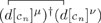and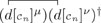with respect to the Fermi vacuum ⟨xΦ|⋯|xΦ⟩, we first expand the transformed operators in terms of the $b̃px$ and $b̃p†x$ operators. The only non-zero contractions between these MO operators are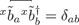and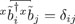. We can therefore expand the contracted product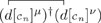as

Inserting the definition of xwZai and $S̃akxw$ from Eqs. (14) and (11), respectively, then leads to the form

Resolving the identity allows us to obtain the additional relationship

$∑a(C̃x)⋅aν⋅ (C̃*x)a⋅⋅σ=gνσ−∑j(C̃x)⋅jν⋅ (C̃*x)j⋅⋅σ,$
(C3)

which, when inserted into Eq. (C2), leads to

To obtain Eq. (C4) from Eq. (C2), we have exploited the relationships

$∑{i|xwS̃i≠0}∑j∑στ(C̃x)⋅jν⋅ (C̃*x)j⋅⋅σ gστ (C̃w)⋅iτ⋅1S̃ixw(C̃*x)i⋅⋅μ=∑{i|xwS̃i≠0}(C̃x)⋅iν⋅(C̃*x)i⋅⋅μ$
(C5)

and

$∑k∈Cn∑j∑στ(C̃x)⋅jν⋅ (C̃*x)j⋅⋅σ gστ (C̃w)⋅kτ⋅(C̃*x)k⋅⋅μ=0.$
(C6)

Finally, Eq. (37a) is recovered by modifying the separation of summation indices in Eq. (C4) to give the form

and introducing the matrices defined in Eqs. (38a)(38d). The second contraction [Eq. (37b)] then follows from

1.
H.
Fukutome
,
Prog. Theor. Phys.
80
,
417
(
1988
).
2.
H.
Koch
and
E.
Dalgaard
,
Chem. Phys. Lett.
212
,
193
(
1993
).
3.
S.
Ten-no
,
Theor. Chem. Acc.
98
,
182
(
1997
).
4.
P. Y.
Ayala
and
H. B.
Schlegel
,
J. Chem. Phys.
108
,
7560
(
1998
).
5.
A. J. W.
Thom
and
M.
,
J. Chem. Phys.
131
,
124113
(
2009
).
6.
E. J.
Sundstrom
and
M.
,
J. Chem. Phys.
140
,
114103
(
2014
).
7.
N. J.
Mayhall
,
P. R.
Horn
,
E. J.
Sundstrom
, and
M.
,
Phys. Chem. Chem. Phys.
16
,
22694
(
2014
).
8.
K. J.
Oosterbaan
,
A. F.
White
, and
M.
,
J. Chem. Phys.
149
,
044116
(
2018
).
9.
K. T.
Jensen
,
R. L.
Benson
,
S.
Cardamone
, and
A. J. W.
Thom
,
J. Chem. Theory Comput.
14
,
4629
(
2018
).
10.
H. G. A.
Burton
and
A. J. W.
Thom
,
J. Chem. Theory Comput.
15
,
4851
(
2019
).
11.
B. C.
Huynh
and
A. J. W.
Thom
,
J. Chem. Theory Comput.
16
,
904
(
2020
).
12.
J.
Nite
and
C. A.
Jiménez-Hoyos
,
J. Chem. Theory Comput.
15
,
5343
(
2019
).
13.
R. K.
Kathir
,
C.
de Graaf
,
R.
Broer
, and
R. W. A.
Havenith
,
J. Chem. Theory Comput.
16
,
2941
(
2020
).
14.
H. G. A.
Burton
and
A. J. W.
Thom
,
J. Chem. Theory Comput.
16
,
5586
(
2020
).
15.
G. E.
Scuseria
,
C. A.
Jiménez-Hoyos
,
T. M.
Henderson
,
K.
Samanta
, and
J. K.
Ellis
,
J. Chem. Phys.
135
,
124108
(
2011
).
16.
T.
Tsuchimochi
and
S.
Ten-no
,
J. Chem. Phys.
144
,
011101
(
2016
).
17.
R.
Dutta
,
G. P.
Chen
,
T. M.
Henderson
, and
G. E.
Scuseria
,
J. Chem. Phys.
154
,
114112
(
2021
).
18.
A. T. B.
Gilbert
,
N. A.
Besley
, and
P. M. W.
Gill
,
J. Phys. Chem. A
112
,
13164
(
2008
).
19.
D.
Hait
and
M.
,
J. Chem. Theory Comput.
16
,
1699
(
2020
).
20.
K.
Carter-Fenk
and
J. M.
Herbert
,
J. Chem. Theory Comput.
16
,
5067
(
2020
).
21.
G.
Levi
,
A. V.
Ivanov
, and
H.
Jónsson
,
224
,
448
(
2020
).
22.
J. A. R.
Shea
and
E.
Neuscamman
,
J. Chem. Phys.
149
,
081101
(
2018
).
23.
T. S.
Hardikar
and
E.
Neuscamman
,
J. Chem. Phys.
153
,
164108
(
2020
).
24.
L. N.
Tran
,
J. A. R.
Shea
, and
E.
Neuscamman
,
J. Chem. Theory Comput.
15
,
4790
(
2019
).
25.
L. N.
Tran
and
E.
Neuscamman
,
J. Phys. Chem. A
124
,
8273
(
2020
).
26.
S. C.
Leasure
and
G. G.
Balint-Kurti
,
Phys. Rev. A
31
,
2107
(
1985
).
27.
J.
Verbeek
and
J. H.
Van Lenthe
,
Int. J. Quantum Chem.
40
,
201
(
1991
).
28.
A.
Igawa
,
Int. J. Quantum Chem.
54
,
235
(
1995
).
29.
Y.
Utsuno
,
N.
Shimizu
,
T.
Otsuka
, and
T.
Abe
,
Comput. Phys. Commun.
184
,
102
(
2013
).
30.
J.
Rodriguez-Laguna
,
L. M.
Robledo
, and
J.
Dukelsky
,
Phys. Rev. A
101
,
012105
(
2020
).
31.
P.-O.
Löwdin
,
Phys. Rev.
97
,
1490
(
1955
).
32.
I.
Mayer
,
Simple Theorems, Proofs, and Derivations in Quantum Chemistry
(
Springer
,
2003
).
33.
A. T.
Amos
and
G. G.
Hall
,
Proc. R. Soc. London, Ser. A
263
,
483
(
1961
).
34.
G. G.
Hall
,
Proc. R. Soc. A
205
,
541
(
1951
).
35.
A.
Szabo
and
N. S.
Ostlund
,
Modern Quantum Chemistry
(
Dover Publications, Inc.
,
1989
).
36.
I.
Shavitt
and
R.
Bartlett
,
Many-Body Methods in Chemistry and Physics
(
Cambridge University Press
,
2009
).
37.
J.
Hendeković
,
M.
Pavlović
, and
F.
Sokolić
,
Chem. Phys. Lett.
77
,
382
(
1981
).
38.
P.
Ring
and
P.
Schuck
,
The Nuclear Many-Body Problem
(
Springer-Verlag
,
1980
).
39.
D. J.
Thouless
,
Nucl. Phys.
21
,
225
(
1960
).
40.
C. A.
Jiménez-Hoyos
,
R.
Rodríguez-Guzmán
, and
G. E.
Scuseria
,
Phys. Rev. A
86
,
052102
(
2012
).
41.
T.
Tsuchimochi
and
S.
Ten-no
,
J. Chem. Theory Comput.
12
,
1741
(
2016
).
42.
S. R.
Yost
,
T.
Kowalczyk
, and
T.
Van Voorhis
,
J. Chem. Phys.
139
,
174104
(
2013
).
43.
S. R.
Yost
and
M.
,
J. Chem. Phys.
145
,
054105
(
2016
).
44.
S. R.
Yost
and
M.
,
J. Chem. Theory Comput.
14
,
4791
(
2019
).
45.
J.
Nite
and
C. A.
Jiménez-Hoyos
, “
Efficient multi-configurational wavefunction method with dynamical correlation using non-orthogonal configuration interaction singles and doubles (NOCISD)
,” chemRxiv (
2019
).
46.
M.
,
P. E.
Maslen
, and
C. A.
White
,
J. Chem. Phys.
108
,
616
(
1998
).
47.
T.
Helgaker
,
P.
Jørgensen
, and
J.
Olsen
,
Molecular Electronic-Structure Theory
(
John Wiley & Sons
,
2000
).
48.
R.
Balian
and
E.
Brezin
,
Nuovo Cimento B
64
,
37
(
1969
).
49.
K. J.
Oosterbaan
,
A. F.
White
, and
M.